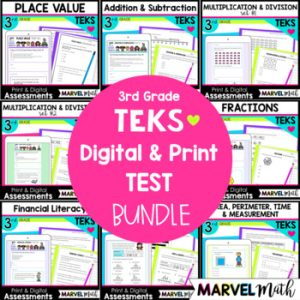Save 10% on your first order with code EXTRA10

# 3rd Grade Area, Perimeter, Time & Measurement Tests – Digital and Print

\$4.00\$18.00
SKU: 7203459 Categories: , ,

## Description

This resource includes 2 ten question Assessments that cover the Measurement TEKS. The questions are STAAR formatted. Test questions cover area, perimeter, elapsed time and measurement. The assessments are in 2 formats: printable PDF and digital, self-grading Google Forms.

The assessments are created to give you meaningful feedback on student progress with all Measurement Content. There are more questions covering the Readiness Standards than the Supporting Standards, as the Readiness Standards are identified as the most critical content for success in subsequent grade levels. Readiness Standards also comprise 2/3 of the STAAR test.

These tests were created with the new STAAR test questions guidelines in mind, and include drop down, multi-select, free response and multiple choice questions.

What’s Included?

• 2 Assessments (Each Test is 10 questions)
• Printable versions of both Assessments
• Color Coded Teacher Key with TEKS identified for each Question

Topics include:

• Area of Rectangles
• Area of Complex (Rectilinear) Figures
• Perimeter of Polygons
• Finding the Missing Side Length in Perimeter problems
• Elapsed Time
• Selecting Units of Measure for Weight and Capacity
• Measuring Weight and Capacity

These tests coordinate with the content covered in this set of Task Cards and these resources work well together!

How Can I Use this Resource?

• Use the 1st test after your Measurement Unit. Based on the results, do targeted reteach and review. Use the 2nd test to re-assess students.
• Use the 1st test after your Measurement Unit. Use the 2nd test as part of your STAAR Review.
• Use the 1st test as a pre-test, use the 2nd test as a post-test.

TEKS Alignment:

3.6C: Determine the area of rectangles with whole number side lengths in problems using multiplication related to the number of rows times the number of unit squares in each row.

3.7B: Determine the perimeter of a polygon or a missing length when given perimeter and remaining side lengths in problems.

Supporting Standards

3.6D: Decompose composite figures formed by rectangles into non-overlapping rectangles to determine the area of the original figure using the additive property of area.

3.7C: Determine the solutions to problems involving addition and subtraction of time intervals in minutes using pictorial models or tools such as a 15-minute event plus a 30-minute event equals 45 minutes.

3.7D: Determine when it is appropriate to use measurements of liquid volume (capacity) or weight.

3.7E: Determine liquid volume (capacity) or weight using appropriate units and tools.